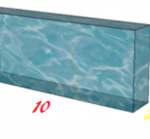Smartick is an online platform for children to master math in only 15 minutes a day

# I want to learn about: Operations & Algebraic Thinking

Learn operations and algebraic thinking in elementary with Smartick. Exercises, theory, tutorials, and teaching resources about operations and algebraic thinking in elementary aged children. Here you will find posts about operations and algebraic thinking to make learning mathematics easier and more fun.

Sep25

## The Associative Property in Addition and MultiplicationAddition and multiplication both use the associative property, while subtraction and division do not. We’re going to to get up close with each situation to get a better idea. The associative property in Addition ♥ Addition indeed has the associative property. Whatever numbers a, b, and c may be, they always end up the same: […]

Sep20

## Introduction to Equations with an Example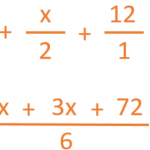In today’s post we’re going to look at the concept of equations. To start, we’re going to take a look at this situation: “One third of Cecilia’s flowerpots contain daisies, half of them are full of pansies, and the other twelve contain poppies. How many flowerpots does Cecilia have?” Think about it, and see if […]

Sep13

## Understanding Multiplication Problems with Examples

What needs to be done in order to solve multiplication problems? Isn’t it enough to just multiply the numbers that we’re given? No! Because… What’s more important is UNDERSTANDING the multiplication problems. This is why we’re bringing you some multiplication problems today where YOU WON’T NEED TO USE MULTIPLICATION, but rather, TO THINK CRITICALLY. Ready? […]

Sep08

## Review the Different Properties of MultiplicationSometimes multiplying can be complicated but knowing the tables and properties of multiplication, we can make this task much easier… let’s get to it! There are three properties of multiplication: commutative, associative, and distributive. Commutative Property It states that the order of the factors does not change the product, that is, it does not matter if […]

Sep01

## How to Solve a Subtraction Problem with Regrouping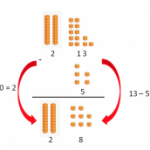Do you know how to solve a subtraction problem with regrouping? Do you know how regrouping is done and why? Today we are going to review how to subtract by regrouping and understand why it is done this way. So that we can picture it better, we are going to do a subtraction with regrouping […]

Aug28

## Multiplying Numbers Followed by Zeros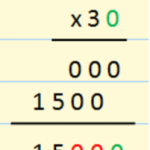In today’s post, we’re going to learn how to multiply numbers that are followed by zeros. You could go about multiplying them like any other number, but we have a trick to teach you, and it’s easy to learn! The most important thing when you learn a trick is to remember why is works the […]

Aug16

## Review Factoring with Examples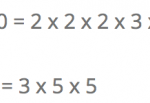Hello again! Do you know what factoring is? Do you know what it is used for? In this post we will answer these questions. How to Factor The factorization of a number is performed by writing the number as the multiplication of all its prime factors. Example: 12 = 2 x 2 x 3 You can […]

Aug11

## Strategy for Mental Calculation: HalvesIn this post we will learn a strategy to divide by 2 (halves), by 4 (fourths) or by 8 (eighths) mentally. We will start by looking at the concept of halving: Divide by Halves: To divide by 2, we just have to look for half of the number. For example, to divide 24/2, we find […]

Aug07

## Divisibility Guidelines for 5 and Some ExamplesIn this post we will learn the criteria of divisibility by 5. A number is divisible by 5 when dividing the number by 5 results in a remainder of zero. But how can we know without having to complete the division? Here we show you the easiest way.   Divisibility criteria for 5: To determine if a number is […]

Jul31

## Mental Math: Multiplying by 25 and 75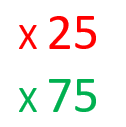In this post we are going to learn a simple way of multiplying by 25 and 75. First, we’ll learn the method and then we’ll give mental math a shot.   Multiplying by 25: The number 25 is 100 divided by 4. So, in order to multiply by 25 what we have to do is […]

Jul24

## Round and Estimate Subtraction ProblemsIn this post, we are going to learn how to round and use estimation in subtraction problems. Mastering this skill is helpful in verifying that an exact answer is of the correct order of magnitude and also in determining whether an answer is reasonable or not. Round and Estimating Subtraction Problems in Tens Round the […]